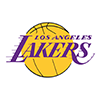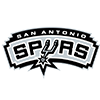PointsBet 2019 Western Conference Odds
+150
1.5 to 1Clippers
33.9% implied probability

+150
1.5 to 1Lakers
33.9% implied probability

+700
7 to 1Rockets
10.6% implied probability

+1200
12 to 1Nuggets
6.5% implied probability

+1200
12 to 1Jazz
6.5% implied probability

+2200
22 to 1Mavericks
3.7% implied probability

+6600
66 to 1Thunder
1.3% implied probability

+8000
80 to 1Trail Blazers
1.0% implied probability

+10000
100 to 1Grizzlies
0.8% implied probability

+10000
100 to 1Pelicans
0.8% implied probability

+25500
255 to 1Spurs
0.3% implied probability

+50000
500 to 1Timberwolves
0.2% implied probability

+50000
500 to 1Suns
0.2% implied probability

+50000
500 to 1Kings
0.2% implied probability

+100000
1000 to 1Warriors
0.1% implied probability